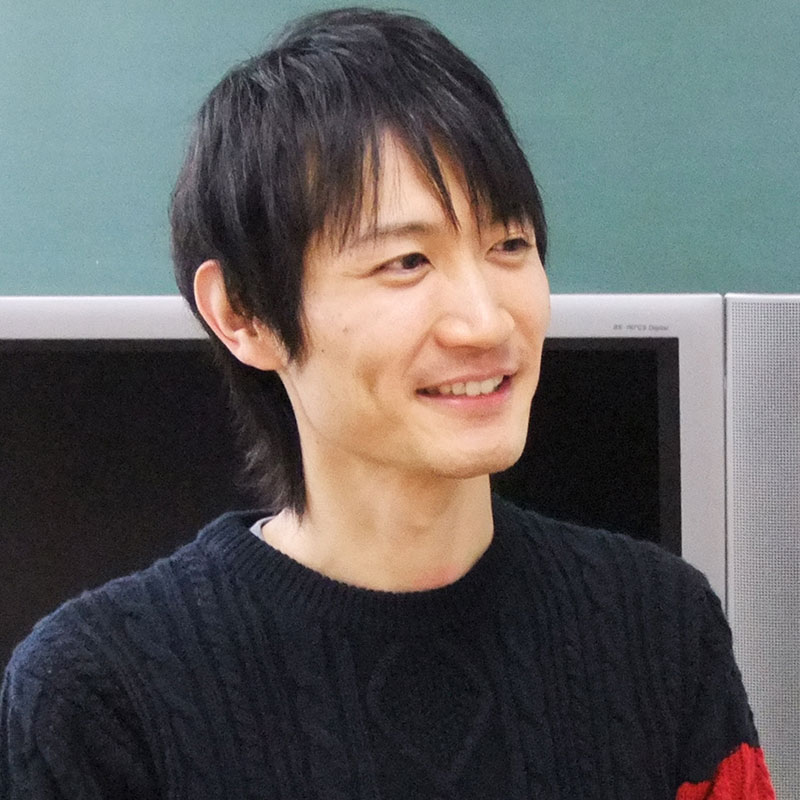## HAMAMUKI NaoAssociate Professor

### Evolving shapes and nonlinear partial differential equations

Department of Mathematics, MathematicsTheme Study of surface evolution equations based on the theory of viscosity solutions Field Partial differential equation Keyword Hamilton-Jacobi equation, Mean curvature flow equation, Maximum principle, Comparison principle, Optimal control, Differential game, Level set method, Viscosity solution

#### Introduction of Research

My major research topic is the study of nonlinear partial differential equations, especially evolution equations such as Hamilton-Jacobi equations and curvature flow equations which appear in materials science and describe a motion of a surface (an interface) separated by two different phases of matter.
On the basis of the theory of viscosity solutions, which is a notion of weak solutions for differential equations, I aim at introducing a suitable notion of solutions, establishing unique existence of solutions to the initial value problem and tracking the asymptotic behavior of solutions to give mathematical foundations to such nonlinear equations.

 Room address Faculty of Science Building #4#### Belongs